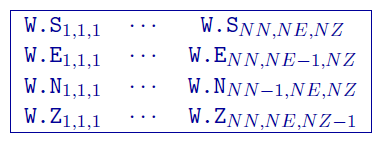# 5.4.6. Weights file¶

This file supplies the user-based weights that acts upon the model objective function. Each set of weights correspond to the functions (e.g., $$w_x$$) given in the model objective function. For ease, the weights in geographic coordinates are provided by the user. The following is the file structure is for the weights file:Parameter definitions:

• W.S$$_{i,j,k}$$: Cell weights for the smallest model component in the model objective function.

• W.E$$_{i,j,k}$$: Cell weights for the interface perpendicular to the easting direction.

• W.N$$_{i,j,k}$$: Cell weights for the interface perpendicular to the northing direction.

• W.S$$_{i,j,k}$$: Cell weights for the interface perpendicular to the vertical direction.

Within each part, the values are ordered in the same way as in model file, however, they can be all on one line, or broken up over several lines. Since the weights for a derivative term are applied to the boundary between cells, the weights have one fewer value in that direction. For instance, the weights for the derivative in easting direction has $$(NE-1) \times NN \times NZ$$ values, whereas the number of cells is $$NE \times NN \times NZ$$.

If the surface is supplied, the cell weights above the surface will be ignored. It is recommended that these weights be assigned a value of -1.0 to avoid confusion. If null is entered instead of the weights file, then all of the cell weights will be set equal (1.0).